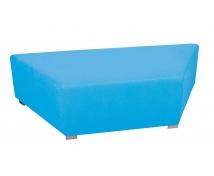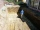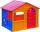# Drainage channel

The cross section of the drainage channel is an isosceles trapezoid whose bases have a length of 1.80 m, 0.90 m and arm has length 0.60 meters. Calculate the depth of the channel.

h =  0.4 m

### Step-by-step explanation:Did you find an error or inaccuracy? Feel free to write us. Thank you!Tips to related online calculators
Pythagorean theorem is the base for the right triangle calculator.

#### You need to know the following knowledge to solve this word math problem:

We encourage you to watch this tutorial video on this math problem:

## Related math problems and questions:Road embankment has a cross-section shape of an isosceles trapezoid with bases 5 m and 7 m, and 2 m long leg. How many cubic meters of soil is in embankment length of 1474 meters?
• EmbankmentPerpendicular cross-section of the embankment around the lake has the shape of an isosceles trapezoid. Calculate the perpendicular cross-section, where bank is 4 m high the upper width is 7 m and the legs are 10 m long.
• DitchDitch profile is an isosceles trapezoid with bases of length 80m and 60m. The slope of the side wall of the ditch is 80°. Calculate the ditch depth.
• Water channelThe cross section of the water channel is a trapezoid. The width of the bottom is 19.7 m, the water surface width is 28.5 m, the side walls have a slope of 67°30' and 61°15'. Calculate how much water flows through the channel in 5 minutes if the water flo
• EstateAn estate-shaped rectangular trapezoid has bases long 34 m, 63 m, and perpendicular arm 37 m. Calculate how long its fence is.
• Land - isosceles trapezoidCalculate the building plot's content and perimeter in the form of an isosceles trapezoid with bases 120m, 95m, and height 50m.
• The ditchDitch with a cross-section of an isosceles trapezoid with bases 2m and 6m are deep 1.5m. How long is the slope of the ditch?
• Isosceles trapezoidFind the area of an isosceles trapezoid, if the bases are 12 cm and 20 cm, the length of the arm is 16 cm
• EmbankmentThe railway embankment 300 m long has a cross-section of an isosceles trapezoid with bases of 14 m and 8 m. The trapezoidal arms are 5 m long. Calculate how much m3 of soil is in the embankment?
• The trenchCalculate how many cubic meters of soil needs to be removed from the excavation in the shape of an isosceles trapezoid, the top width is 3 meters, the lower width is 1.8 m, the depth of the excavation is 1 m, and the length is 20 m.
• Isosceles trapezoidCalculate the area of an isosceles trapezoid whose bases are in the ratio of 4:3; leg b = 13 cm and height = 12 cm.
• The gardenThe garden has the shape of a rectangular trapezium. The bases have lengths of 27 meters and 36 meters, the trapezoid's height is 12 meters. Calculate how much fence will cost this garden if one meter costs 1.5 €?
• Isosceles trapezoidCalculate the content of an isosceles trapezoid whose bases are at ratio 5:3, the arm is 6cm long and it is 4cm high.
• IS trapezoidCalculate the length of diagonal u and height v of isosceles trapezoid ABCD, whose bases have lengths a = |AB| = 37 cm, c = |CD| = 29 cm and legs b = d = |BC| = |AD| = 28 cm.
• Face of the houseHow tall is the gable of a house in the shape of an isosceles triangle with a base length of 8 meters and an arm 5 meters long?
• Isosceles trapezoidCalculate the circumference and the contents of the isosceles trapezoid if you know the size of the bases is 8 and 12 cm and the size of the arms is 5 cm.
• Railway embankmentThe railway embankment section is an isosceles trapezoid, the sizes of the bases of which are in the ratio 5: 3. The arms have a length of 5 m, and the height of the embankment is 4.8 m. Calculates the size of the embankment section area.